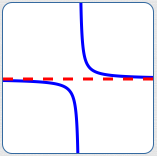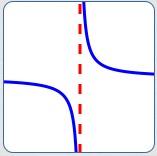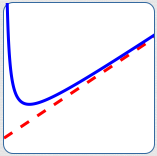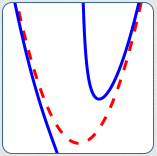﻿ Introduction to Asymptotes

# INTRODUCTION TO ASYMPTOTES

• PRACTICE (online exercises and printable worksheets)

DEFINITION asymptote
An ‘asymptote’ (pronounced AS-sim-tote) is a curve (usually a line)
that another curve gets arbitrarily close to as $\,x\,$ approaches $\,+\infty\,$, $\,-\infty\,$, or a finite number.

Rational functions usually have interesting asymptote behavior.

Asymptotes exhibited by rational functions come in different flavors, as shown below:horizontal asymptote The dashed red line is horizontal. The blue curve is getting closer and closer to this horizontal red line as $\,x\rightarrow\infty\,$ and as $\,x\rightarrow -\infty\,$. Thus, the red line is a horizontal asymptote.vertical asymptote The dashed red line is vertical. The blue curve is getting closer and closer to this vertical red line as $\,x\,$ approaches a finite number (from the right, and from the left). Thus, the red line is a vertical asymptote.slant asymptote The dashed red line is not horizontal, and not vertical. It is ‘slanted’. The blue curve is getting closer and closer to this ‘slanted’ red line as $\,x\rightarrow\infty\,$. Thus, the red line is a slant asymptote.asymptotes that are not lines The dashed red curve is not a line. The blue curve is getting closer and closer to this red curve as $\,x\rightarrow\infty\,$ and as $\,x\rightarrow -\infty\,$. Thus, the red curve is an asymptote that is not a line.

Vertical, horizontal and slant asymptotes are studied in more detail in the next few sections.

Master the ideas from this section